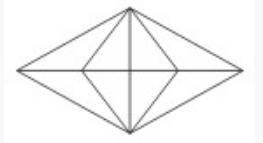Courses

# How many triangles are there in the following figure?a)10b)14c)22d)20Correct answer is option 'D'. Can you explain this answer? Related Test: SSC CPO Mock Test (GI & Reas) 1

## SSC Question

By Rahul Yadav · 3 weeks ago ·SSC
There are eight triangles in the upper half of the figure, namely - ABE, AEO, ABO, AOF, AFC, AOC, AEF and ABC

Similarly, there are eight triangles in the lower half of the figure
There are four more triangles, namely : ABD, AED, AFD and ACD
Hence there are a total of twenty triangles in the figure.

Akansha Gupta answered 2 weeks ago
D

Miss Ruby answered 2 days ago
3*4+3+3+2=20